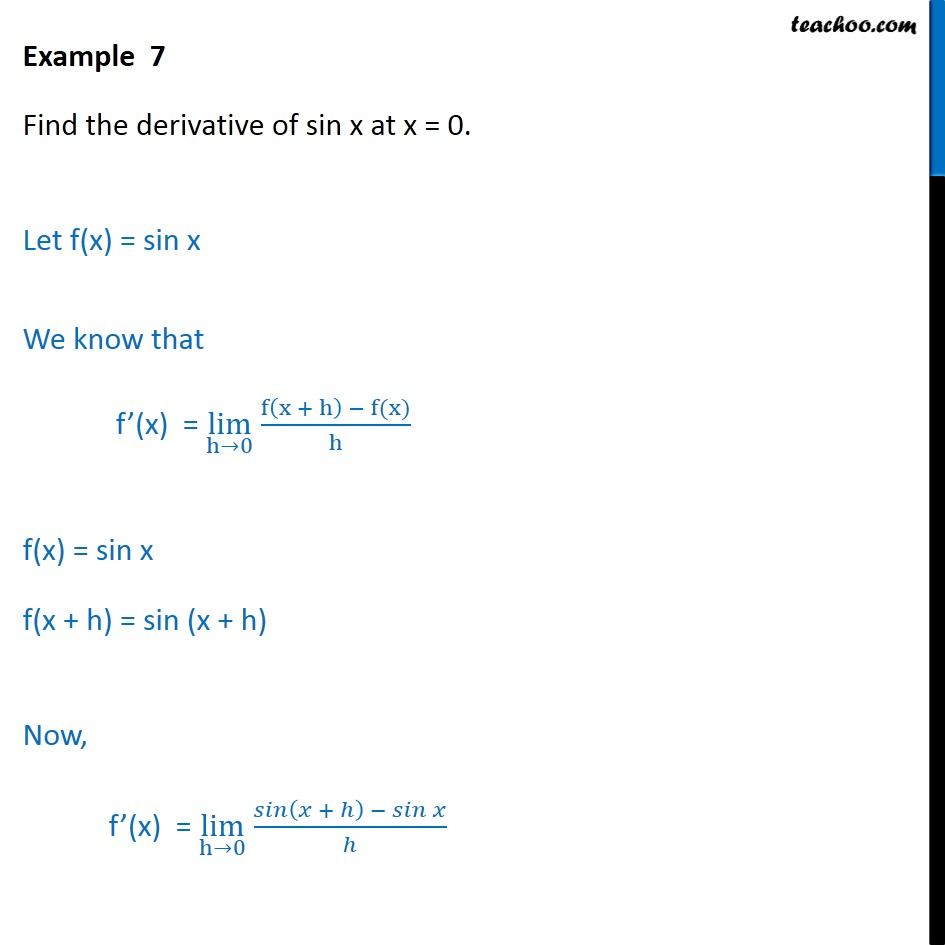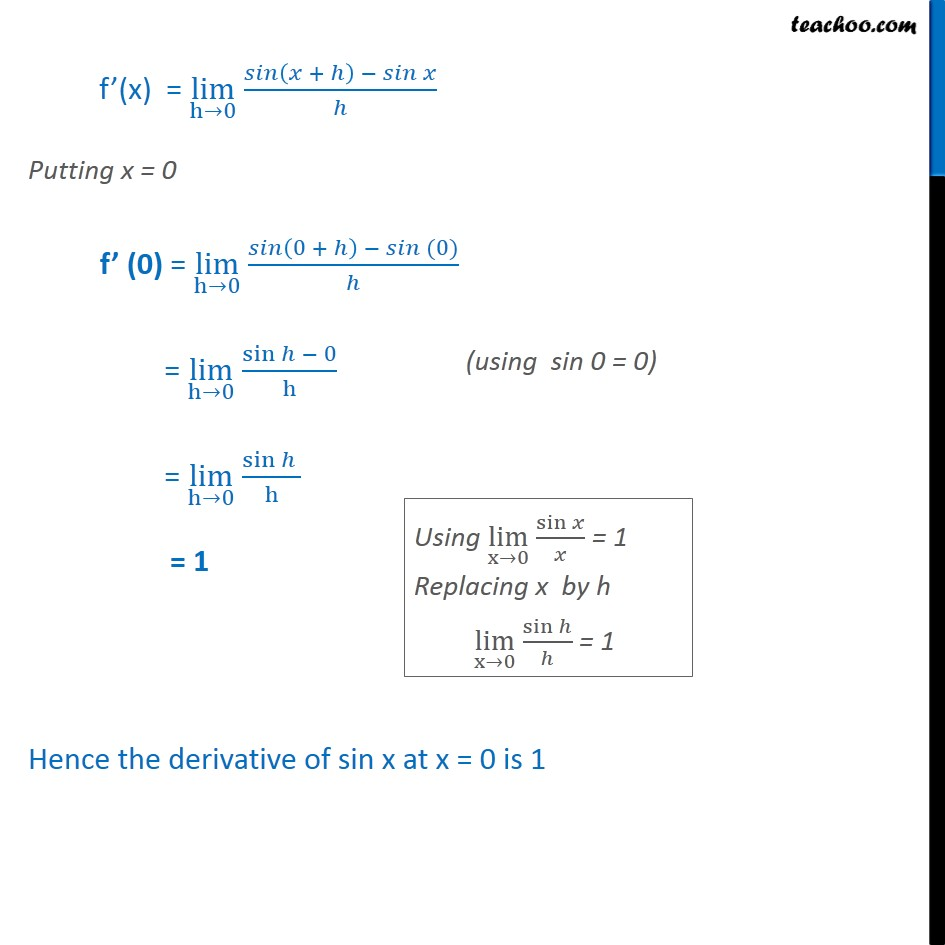1. Chapter 13 Class 11 Limits and Derivatives
2. Serial order wise
3. Examples

Transcript

Example ,7 Find the derivative of sin x at x = 0. Let f(x) = sin x We know that f’(x) = lim﷮h→0﷯ f﷮ x + h﷯ − f(x)﷯﷮h﷯ f(x) = sin x f(x + h) = sin (x + h) Now, f’(x) = lim﷮h→0﷯ 𝑠𝑖𝑛﷮ 𝑥 + ℎ﷯ − 𝑠𝑖𝑛 𝑥﷯﷮ℎ﷯ f’(x) = lim﷮h→0﷯ 𝑠𝑖𝑛﷮ 𝑥 + ℎ﷯ − 𝑠𝑖𝑛 𝑥﷯﷮ℎ﷯ Putting x = 0 f’ (0) = lim﷮h→0﷯ 𝑠𝑖𝑛﷮ 0 + ℎ﷯ − 𝑠𝑖𝑛 (0)﷯﷮ℎ﷯ = lim﷮h→0﷯ sin﷮ℎ − 0﷯﷮h﷯ = lim﷮h→0﷯ sin﷮ℎ ﷯﷮h﷯ = 1 Hence the derivative of sin x at x = 0 is 1

Examples

About the AuthorDavneet Singh
Davneet Singh is a graduate from Indian Institute of Technology, Kanpur. He has been teaching from the past 9 years. He provides courses for Maths and Science at Teachoo.